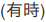# Scalable Data Science

### Course Project by Akinwande Atanda

The html source url of this databricks notebook and its recorded Uji:#Tweet Analytics

## Creating Pipeline with Loop and Productionizing with Historical Tweets

``````
from pyspark.ml import *
from pyspark.ml import Pipeline
from pyspark.ml.feature import *
from pyspark.ml.classification import *
from pyspark.ml.tuning import *
from pyspark.ml.evaluation import *
from pyspark.ml.regression import *
from pyspark.sql.types import *

``````
``````
df = table("pos_neg_category")

``````
``````
df.dtypes

``````
``````
lrARValidate =[]
lrARTest =[]
param = [0.0,0.1,0.2,0.3,0.4,0.5,0.6,0.7,0.8,0.9,1.0]
for p in param:
bin = Binarizer(inputCol = "category", outputCol = "label", threshold = 0.5) # Positive reviews > 0.5 threshold
tok = Tokenizer(inputCol = "review", outputCol = "word") #Note: The column "words" in the original table can also contain sentences that will be tokenized
hashTF = HashingTF(inputCol = tok.getOutputCol(), numFeatures = 5000, outputCol = "features")
lr = LogisticRegression(maxIter = 10, regParam = 0.01, elasticNetParam = p)
pipeline = Pipeline(stages = [bin, tok, hashTF, lr])
(trainingData, validateData, testData) = df.randomSplit([0.6, 0.3, 0.1])
model = pipeline.fit(trainingData)
validateModel=model.transform(validateData)
evaluator = MulticlassClassificationEvaluator(labelCol="label", predictionCol="prediction", metricName="precision")
accuracyValidateSet = evaluator.evaluate(validateModel)
testModel=model.transform(testData)
accuracyTestSet = evaluator.evaluate(testModel)
#   print("Logistic Regression Classifier Accuracy Rate for Validation Dataset = %g " % (accuracyValidateSet))
#   print("Logistic Regression Classifier Accuracy Rate for Test Dataset = %g " % (accuracyTestSet))
#   print("Test Error = %g " % (1.0 - accuracy))
lrARValidate +=[(p,accuracyValidateSet)]
lrARTest +=[(p,accuracyTestSet)]

``````
``````
#display(pipeline)

``````
``````
lrARValidate

``````
``````
lrARTest

``````
``````
print("Logistic Regression Classifier Accuracy Rate for Validation Dataset= ", lrARValidate)

``````
``````
print("Logistic Regression Classifier Accuracy Rate for Test Dataset= ", lrARTest)

``````

## Productionizing with Historical Tweets

``````

``````

Convert to Table

``````
trumpTweet.registerTempTable('TrumpTweetTable')

``````
``````

``````

Read the data type of each column in the table

``````
trumpTweet.dtypes

``````
``````
# sqlContext.sql("SELECT COUNT(*) FROM TrumpTweetTable")

``````

Change the favourite count from double to float

``````
sqlContext.sql("SELECT date, review, CAST(category as FLOAT) as category FROM TrumpTweetTable order by date asc").cache

``````

Randomly split Dataframe into two or three sets

``````
(trump1, trump2, trump3) = trumpTweet.randomSplit([0.1, 0.5, 0.4])

``````

Transform the fitted algorithm to predict the category of the tweet being either positive or negative

``````
#  tweetModel=model.transform(trump1)

``````

Transform the fitted algorithm to predict the category of the tweet being either positive or negative

``````
tweetModel=model.transform(trumpTweet)

``````

Determine the accuracy rate of the predicted sentiment

``````
evaluator = MulticlassClassificationEvaluator(labelCol="label", predictionCol="prediction", metricName="precision")

``````
``````
accuracytweetSet = evaluator.evaluate(tweetModel)

``````
``````
accuracytweetSet

``````
``````
# display(tweetModel.select("prediction", "review", "probability"))

``````

Display the predicted category, tweet and probability of the tweet being negative

``````
tweetModel.select("prediction", "review", "probability").show(100)

``````

Save the sentiment category of the historical tweets for additional ETL

``````
trumpSentiment=tweetModel.select("prediction", "review", "probability")

``````
``````
trumpSentiment.write.save("dbfs:/mnt/s3Data/trumpSen.parquet")

``````
``````
trumpSentiment.show(50)

``````
``````
display(dbutils.fs.ls("dbfs:/mnt/s3Data"))

``````
``````

``````
``````
trumpSen.registerTempTable('trumpSenTable')

``````
``````
%sql SELECT COUNT(*) as TweetCount FROM trumpSenTable

``````
``````
%sql SELECT * FROM trumpSenTable WHERE prediction ==1 LIMIT 5

``````

Count and plot the percentage of Tweets about Trump that is positive and negative

``````
%sql SELECT if(prediction == 1, "positive", "negative") as Sentiment, count(*) as TweetCount FROM trumpSenTable GROUP BY prediction ORDER BY prediction

``````

Updated: